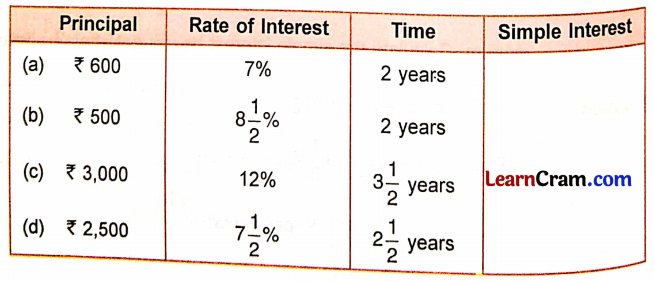# DAV Class 5 Maths Chapter 13 Worksheet 2 Solutions

The DAV Books Solutions Class 5 Maths and DAV Class 5 Maths Chapter 13 Worksheet 2 Solutions of Simple Interest offer comprehensive answers to textbook questions.

## DAV Class 5 Maths Ch 13 Worksheet 2 Solutions

Question 1.
Find the simple interest at the end of one year for the following:
(a) ₹ 400 at 5% interest per annum.
Solution:
5% = $$\frac{5}{100}$$ means ₹ 5 interest for every ₹ 100 deposited.
Simple interest on ₹ 1 for one year = ₹ $$\frac{5}{100}$$
Simple interest on ₹ 400 for one year = $$\frac{5}{100}$$ × 400 = ₹ 20

(b) ₹ 1,800 at 12% interest per annum.
Solution:
12% = $$\frac{12}{100}$$ means ₹ 12 interest for every ₹ 100 deposited
Simple interest on ₹ 1 for one year = ₹ $$\frac{12}{100}$$
Simple interest on ₹ 1,800 for one year = $$\frac{12}{100}$$ × 1800 = ₹ 216(c) ₹ 2,500 at 4$$\frac{1}{2}$$% interest per annum.
Solution:
4$$\frac{1}{2}$$% = $$\frac{9}{2}$$% = $$\frac{9}{200}$$ means ₹ 9 interest for every ₹ 200 deposited
Simple interest on ₹ 1 for one year = ₹ $$\frac{9}{200}$$
Simple interest on ₹ 2,500 for one year = ₹ $$\frac{9}{200}$$ × 2500
= ₹ $$\frac{225}{2}$$
= ₹ 112.5

(d) ₹ 3,200 at 6$$\frac{1}{2}$$% interest per annum.
Solution:
6$$\frac{1}{2}$$% = $$\frac{13}{2}$$% = $$\frac{13}{200}$$ means ₹ 13 interest for every ₹ 200 deposited
Simple interest on ₹ 1 for one year = ₹ $$\frac{13}{200}$$
Simple interest on ₹ 3,200 for one year = ₹ $$\frac{13}{200}$$ × 3200 = ₹ 208

Question 2.
Complete the table by calculating the simple interest.Solution:
(a) P = ₹ 600, R = 7% per annum, T = 2 years
Simple Interest = P × R × T
= 600 × $$\frac{7}{100}$$ × 2
= ₹ 84

(b) P = ₹ 500, R = 8$$\frac{1}{2}$$% = $$\frac{17}{2}$$% = $$\frac{17}{200}$$ per annum, T = 2 years
Simple Interest = P × R × T
= 500 × $$\frac{17}{200}$$ × 2
= ₹ 85(c) P = ₹ 3,000, R = 12% = $$\frac{12}{100}$$ per annum, T = 3$$\frac{1}{2}$$ years = $$\frac{7}{2}$$ years
Simple interest = P × R × T
= 3,000 × $$\frac{12}{100} \times \frac{7}{2}$$
= ₹ 1,260

(d) P = ₹ 2,500, R = 7$$\frac{1}{2}$$% = $$\frac{15}{2}$$% = $$\frac{15}{200}$$ per annum, T = 2$$\frac{1}{2}$$ years = $$\frac{5}{2}$$ years
Simple interest = P × R × T
= 2,500 × $$\frac{15}{200} \times \frac{5}{2}$$
= $$\frac{1875}{4}$$
= ₹ 468.75

Question 3.
Solve the following questions:
(a) A man deposited ₹ 4,500 in a bank which paid him an interest of 11% per annum. How much simple interest will he get at the end of five years?
Solution:
Principal = ₹ 4,500
Rate of interest = 11% per annum = $$\frac{11}{100}$$ per annum
Time = 5 years
SI = P × R × T
= $$\frac{4500 \times 11 \times 5}{100}$$
= ₹ 2475
He will get a simple interest of ₹ 2475 at the end of five years.

(b) Rahul borrowed ₹ 650 from Sohan at 8% interest per annum. Find the simple interest Rahul has to pay Sohan after 4$$\frac{1}{2}$$ years.
Solution:
Principal = ₹ 650
Rate of interest = 8% per annum = $$\frac{8}{100}$$ per annum
Time = 4$$\frac{1}{2}$$ years = $$\frac{9}{2}$$ years
SI = P × R × T
= 650 × $$\frac{8}{100} \times \frac{9}{2}$$
= $$\frac{13 \times 4 \times 9}{2}$$
= ₹ 234
Rahul has to pay simple interest of ₹ 234 to Sohan after 4$$\frac{1}{2}$$ years.(c) Ramlal borrowed ₹ 3,000 from Arun to purchase a tractor. Arun charged him an interest of 4$$\frac{1}{2}$$% per annum. How much simple interest will Ramlal pay to Arun after three years?
Solution:
Principal = ₹ 3,000
Rate of interest = 4$$\frac{1}{2}$$% = $$\frac{9}{2}$$% = $$\frac{9}{200}$$ per annum
Time = 3 years
SI = P × R × T
= 3,000 × $$\frac{9}{200}$$ × 3
= 15 × 27
= ₹ 405
Ramlal has to pay a simple interest of ₹ 405 to Arun after three years.

DAV Class 5 Maths Chapter 13 Worksheet 2 Notes

Simple Interest
The calculation of simple interest depends on three factors:

• Principal
• Rate of Interest
• Time

Example 1.
Calculate the simple interest on ₹ 600 at 12% interest per year.
Solution:
Simple interest on ₹ 100 for one year = ₹ 12
Simple interest on ₹ 1 for one year = ₹ $$\frac{12}{100}$$
Simple interest on ₹ 600 for one year = ₹ $$\frac{12}{100}$$ × 600 = ₹ 72
So, the interest at the end of one year will be ₹ 72.Simple Interest = Principal × Rate of Interest × Time
SI = P × R × T

Example 2.
Mr. Sushil deposited ₹ 900 in a bank at 3$$\frac{1}{2}$$% rate of interest per annum. Calculate the simple interest he will get after 2$$\frac{1}{2}$$ years.
Solution:
Principal = ₹ 900
Rate of interest = 3$$\frac{1}{2}$$% per annum
= $$\frac{7}{2}$$% per annum 2
= $$\frac{7}{200}$$ per annum
Time = 2$$\frac{1}{2}$$ years = $$\frac{5}{2}$$ years
Simple Interest = P × R × T
= 900 × $$\frac{7}{200} \times \frac{5}{2}$$
= $$\frac{315}{4}$$
= ₹ 78.75